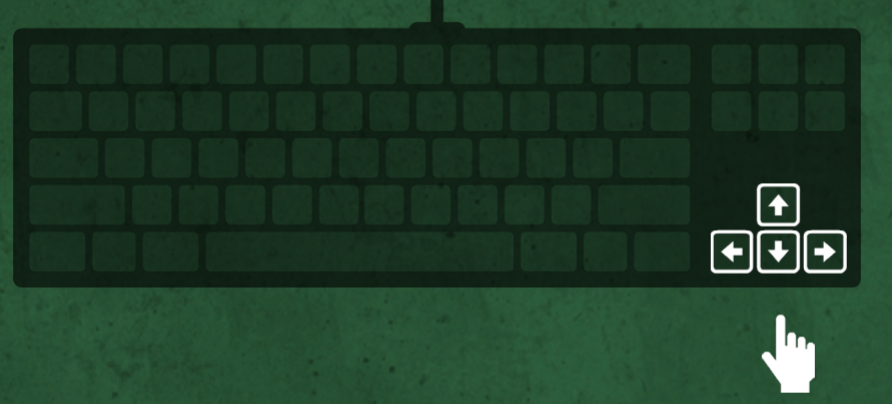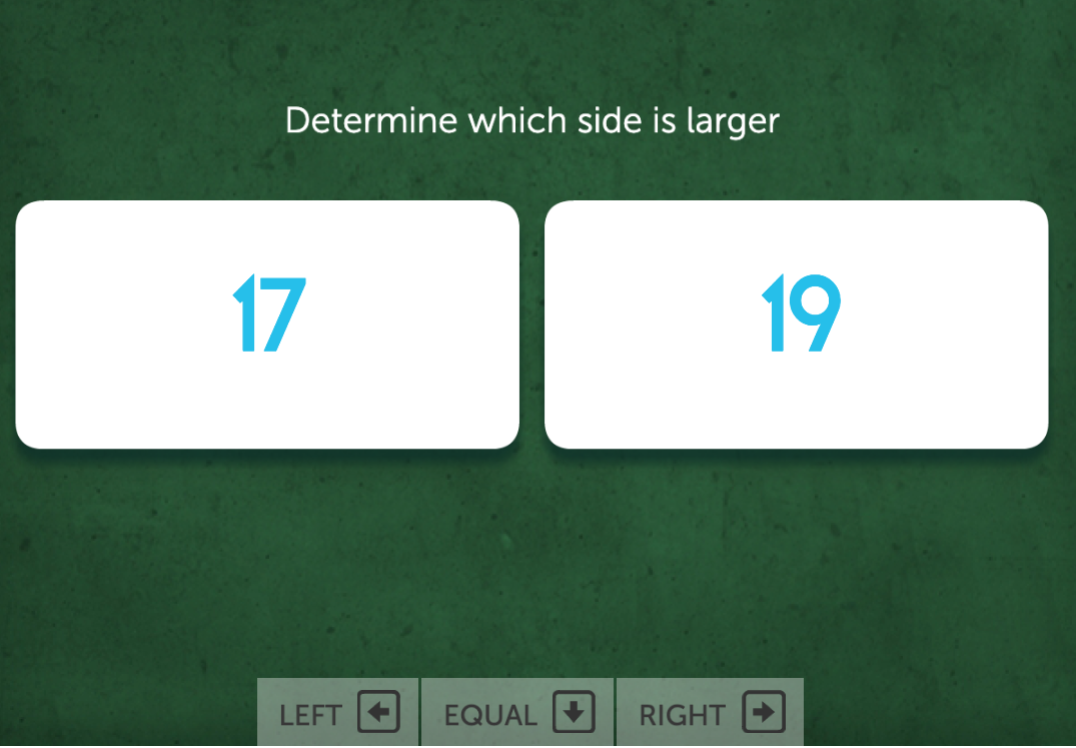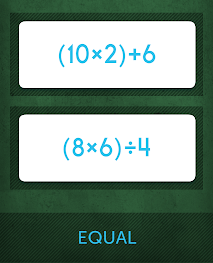# Chalkboard Challenge instructions

In Chalkboard Challenge, you are given two equations. You need to determine which side is larger

This game uses the keyboard arrow keys to key in the answers.Tap either the left or right arrows and tap the down arrow if the sides are equal.When playing on mobile, tap on the larger equation. Tap either top, bottom, or equal (if both cards are equal.)You can review instructions for any of our games at any time. On a computer, click How to Play before starting the game. Or, during the game, clicking the pause (II) button in the top-left corner and then selecting How to Play.

In the app, then tap the question mark (?) to the left of the Play button before starting. Or, during the game, tap pause (II) at the top-left of the screen and then tap How To Play from the menu that appears.

## Scoring & Levels

Chalkboard Challenge uses metered scoring to calculate your final score. There is a meter at the top of the game screen that fills by 1 when you get a trial correct. If the meter fills to 4, the multiplier increases (up to a maximum of 10), and the meter is reset.

For example, if you get the first 4 answers correct in a row, the meter fills up and then your multiplier increases to 2. The meter then also empties and it begins to fill once again. At the multiplier level of 1, you get 50 points for every correct answer. Once your multiplier increases, you get 50 times your multiplier level for each correct answer. So at multiplier level 4, you earn 200 points for each correct answer. The highest multiplier you can get is 10.

If you get a trial incorrect and the meter is partially filled, then the meter resets to empty. If you get an answer wrong when the meter is already empty, your multiplier then decreases by 1. Your overall score depends on when you got an answer. This affects how many points you get for your next correct answers.

For example, say you are at multiplier level 4 and your meter is filled up by 3. If you get the next answer correct, you earn 200 points and your multiplier increases to 5. This means your next correct answer earns you 250 points.

If you get the answer incorrect, however, you do not earn any points. Your meter also returns to empty. This means your next correct answer only earns you 200 points, and you have to get 4 correct answers in a row again to earn 250 points per answer.

You also earn a final bonus depending on the multiplier level you finish with at the end of the game. You earn 250 points times your multiplier level. If you're at level 10 (the highest level) when the game ends, you then earn a bonus of 2,500 points. Your final score also depends on what level you finish with at the end of the game.

Last Updated: